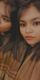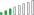cancel
Showing results for
Did you mean:New Member

## Percentage of Row Totals to display 0% for a row with no data in matrix visual

I am trying to get a matrix to provide bookings and percentage of bookings (per day) in a given week. However, some weeks have no bookings and so when I use the values to give %RT, it leaves it blank as the total is 0. I need it to display 0% for each day instead of blank values.

I have tried to calculate %RT as a DAX measure as well, but it just filters out and does not give 0%. Is there any way I can use custom string field or other methods.

I hope someone can help me find a solution.1 ACCEPTED SOLUTIONResolver III

You will need to write it...

``````Measure =
VAR currentTotal = [Bookings]
VAR WeekDayTotal = CALCULATE([Bookings],ALLSELECTED(WeekDayName))
VAR Result = DIVIDE(currentTotal,WeekDayTotal,0)
RETURN IF(ISBLANK(Result),0,Result)``````Resolver III

You will need to write it...

``````Measure =
VAR currentTotal = [Bookings]
VAR WeekDayTotal = CALCULATE([Bookings],ALLSELECTED(WeekDayName))
VAR Result = DIVIDE(currentTotal,WeekDayTotal,0)
RETURN IF(ISBLANK(Result),0,Result)``````